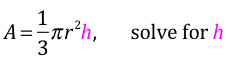Literal Equations and Dimensional Analysis
Algebra
After watching the Yay Math video on this concept, take this quiz to see how much you've learned! See the results right when you're done, AND, there's even an option to send the results to an email of your choice. Good luck, no pressure, and YAY MATH!

Would you like to share this quiz with others who, like you, envision a world where people love math? Let's get started. Here is the shortened link: https://forms.gle/RgAgo4tuD7bdyPpm9
Email send?
(If you want your score automatically sent to you or to someone else, which email would you like to use? This is optional and for your convenience; everything is kept private.)
Please solve the following equations, for the specified variable.
y = m – a (solve for m) *
1 point
y = m – a (solve for a) *
1 point
ab = c + d (solve for a) *
1 point
x = 4 + c/y (for c) *
1 point3m + 2c = m + 6 (for m) *
1 point
bx = cx + m (for x) *
(Hint: get all the x values on the left, then factor x out. In other words, do the Distributive Property, except in reverse order.)
1 point
The formula for the volume of a cone is A = ⅓πr²h. Please solve for h. *
1 point